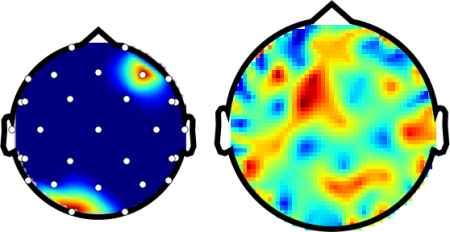# Generating Topography plots¶

Example demonstrating a topography plot.

```from mvpa2.suite import *

# Sanity check if we have griddata available
externals.exists("griddata", raise_=True)

# EEG example splot
pl.subplot(1, 2, 1)

# load the sensor information from their definition file.
# This file has sensor names, as well as their 3D coordinates
sensors=XAVRSensorLocations(os.path.join(pymvpa_dataroot, 'xavr1010.dat'))

# make up some artifical topography
# 'enable' to channels, all others set to off ;-)
topo = np.zeros(len(sensors.names))
topo[sensors.names.index('O1')] = 1
topo[sensors.names.index('F4')] = 1

# plot with sensor locations shown
plot_head_topography(topo, sensors.locations(), plotsensors=True)

# MEG example plot
pl.subplot(1, 2, 2)

# load MEG sensor locations
sensors=TuebingenMEGSensorLocations(
os.path.join(pymvpa_dataroot, 'tueb_meg_coord.xyz'))

# random values this time
topo = np.random.randn(len(sensors.names))

# plot without additional interpolation
plot_head_topography(topo, sensors.locations(),
interpolation='nearest')
```

The ouput of the provided example should look likeSee also

The full source code of this example is included in the PyMVPA source distribution (`doc/examples/topo_plot.py`).